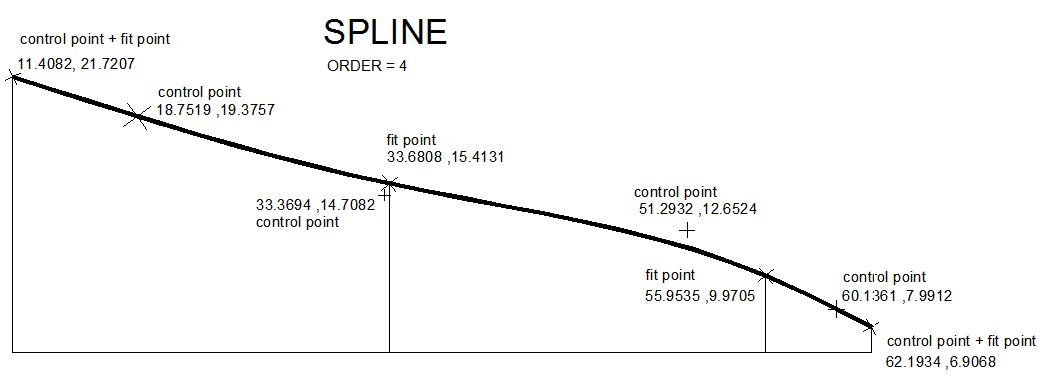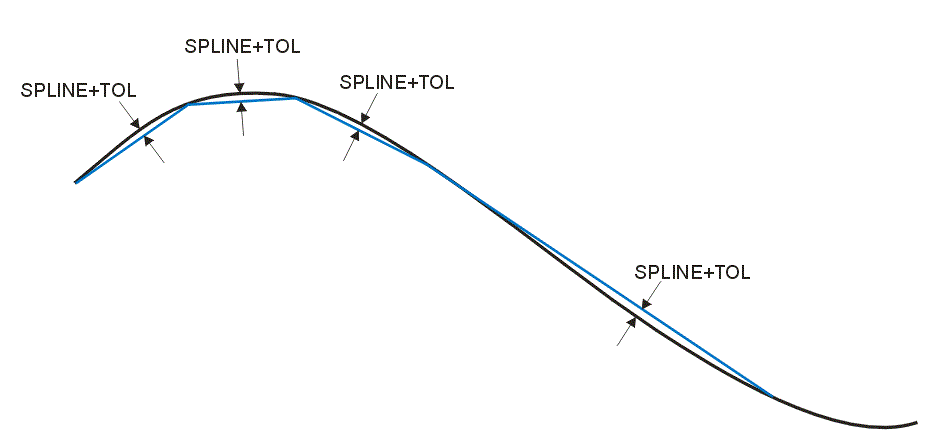## Spline Conversion to Segments

In addition to arcs and lines, AutoCAD supports splines. These are not normally used for printed circuit boards or integrated circuit design with the exception of optical components where a tapered waveguide may use a spline in the boundary. DXF2GDS supports the conversion of splines into multiple segments and the user has control over the precision of the approximation.

Per Autodesk

"A spline is a smooth curve that passes through or near a set of points that influence the shape of the curve.

By default, a spline is a series of blended curve segments of degree 3 (also called cubic) polynomials. These curves are technically called nonuniform rational B-splines (NURBS), but are referred to as splines for simplicity. Cubic splines are the most common, and mimic the splines that are created manually using flexible strips that are shaped by weights at data points."

A spline drawn in AutoCAD is shown below along with the control points and fit points.### Parameters for Spline Segmentation

Two parameters control the segmentation of splines: SPLINE_RULE and SPLINE_TOLERANCE. There are three values of SPINE_RUL: 1,2,3. Each of the rules results in different behavior. If SPLINE_TOL is not set, or is set to a large value, the ARCSAG parameter may be used in lieu of SPINE_TOL.

SPLINE_RULE=1

SPLINE_TOL is ignored. The total number of segments is equal to 9X the number of knots.

SPLINE_RULE=2

The value of SPLINE_TOL will be used to control smoothness. If SPLINE_TOL=0, then the ARCSAG value will be used. If both SPLINE_TOL=0 and ARCSAGE=0, then the behavior reverts to SPLINE_RULE=1. The smoothness of the of the contour between knot values will be on the order of the tolerance value, i.e. the length of each approximating segment will be close to the tolerance value. Because spline data represent nonlinear parametric curves not all approximating GDSII segment lengths will be the same length.

SPLINE_RULE=3

SPLINE_RULE=3 is similar to SPLINE_RULE=2; as the segments are generated their number and length are selected in an attempt to conform to a rule analogous to how ARCs and CIRCLEs are segmented. Specifically the chord error between the idealized curve and the approximating segments will not exceed the value associated with SPLINE_TOL.If the value for SPLINE_TOL is greater than 3 times the value of ARCSAG or it is zero, then the tolerance will be set to 3 times the value of ARCSAG. If both the value of SPLINE_TOL is zero and the value of ARCSAG is zero then SPLINE_RULE=1 is used.

 SPLINE_RULE